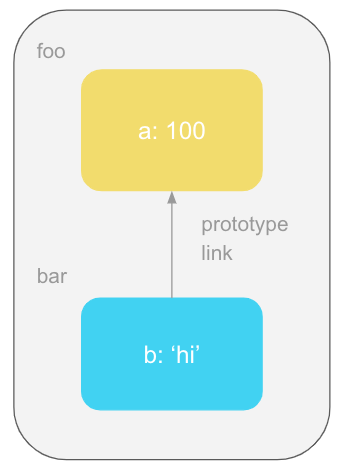# 你懂 JavaScript 嗎？#3 暖身 (๑•̀ㅂ•́)و✧ Part 2 - 變數、嚴格模式、IIFEs、閉包、模組、this、原型、Polyfill 與 Transpiler• 變數的存取規則，包含函式範疇拉升巢狀範疇
• 嚴格模式：一個讓程式碼變得更好、更容易優化的方法。
• 更多關於範疇和函式的應用，包含 IIFE閉包模組
• this：到底是指哪個？這個還是那個？應該不少人都黑人問號 XD
• 原型可說是物件的一種 fallback 機制，並且提供了行為委派。
• 舊功能與新特色的共存，可使用 PolyfillTranspiler 來做兼容。## 變數（Variable）

### 函式範疇（Function Scope）

``````function foo(a) {
var b = 2;

function bar() {
// ...
}

var c = 3;
}

console.log(a); // ReferrenceError
console.log(b); // ReferrenceError
console.log(c); // ReferrenceError

bar(); // ReferrenceError
``````

### 拉升（Hoisting）

``````var a; // 編譯時期的工作

console.log(a); // undefined
a = 2; // 執行時期的工作
``````

### 巢狀範疇（Nested Scope）

``````const foo = (a) => {
console.log(a + b);
};

const b = 2;

foo(2); // 4
``````

## 嚴格模式（Strict Mode）

``````'use strict';

a = 1; // Uncaught ReferenceError: a is not defined
``````## 作為值的函式（Function as Value）

``````var foo = function () {
console.log('大家好，我是 foo！');
};
``````

``````var foo = function () {
console.log('大家好，我是 foo！');
};

function bar(func) {
func();
}

bar(foo); // 大家好，我是 foo！
``````

``````var foo = function () {
console.log('大家好，我是 foo！');
};

var result = function baz(func) {
return func;
};

result(foo)(); // 大家好，我是 foo！
``````### 即刻調用函式運算式（Immediately Invoked Function Expression, IIFE）

IIFE 是為可立即執行的函式運算式。一般的函式運算式並不會馬上執行，若要執行除了在其名稱後加上小括號外，還可以利用 IIFE 的方式執行它，匿名或具名皆合法。使用 IIFE 的好處主要是不污染全域範疇。

``````(function () {
var a = 3;
console.log(a); // 3
})();

// 不污染全域範疇
a; // Uncaught ReferenceError: a is not defined
``````

### 閉包（Closure）

``````function closure() {
var a = 1;
console.log(a); // 1
}

closure();
a; // Uncaught ReferenceError: a is not defined
``````

### 模組（Module）

``````function CoolModule() {
var something = 'cool';
var another = [1, 2, 3];

function doSomething() {
console.log(something);
}

function doAnother() {
console.log(another.join(' ! '));
}

return {
doSomething: doSomething,
doAnother: doAnother,
};
}

var foo = CoolModule();

foo.doSomething(); // cool
foo.doAnother(); // 1 ! 2 ! 3
``````

## this 識別字（this Identifier）

this 到底是指向誰一直都是個令人費解的問題。• new 綁定：this 會指向 new 出來的物件。
• 明確綁定：使用 call、apply、bind，明確指出要綁定給 this 的物件。
• 隱含綁定：當函式為物件的方法（method）時，在執行階段 this 就會被綁定至該物件。
• 預設綁定：當其他規則都不適用時，意即沒有使用 bind、call、apply 或不屬於任何物件的 method，就套用預設綁定，在非嚴格模式下，瀏覽器環境 this 的值就是預設值全域物件 window，而在嚴格模式下，this 的值就是 undefined。

``````function foo() {
console.log(this.bar);
}

var bar = 'global';

var obj1 = {
bar: 'obj1',
foo: foo,
};

var obj2 = {
bar: 'obj2',
};

foo(); // 'global'
obj1.foo(); // 'obj1'
foo.call(obj2); // 'obj2'
new foo(); // undefined
``````

## 原型（Prototype）

``````var foo = { a: 100 };

var bar = Object.create(foo); // 建立 bar 物件，並連結到 foo
bar.b = 'hi';

bar.a; // 100，委派給 foo
bar.b; // 'hi'
``````## 舊功能與新特色的共存

### Polyfill

Polyfilling 的意思就是依據一個新功能的定義，製作具有相同行為，而能在較舊的 JavaScript 環境執行的程式碼，白話說就是為舊瀏覽器掛載新功能。

#### isNaN

NaN 表示值為無效的數字，它會產生的原因是

• 做數學運算時的兩個運算元的資料型別並非都數字或無法轉成有效的十進位或十六進位的數字。
• 無意義的運算，例如：0 / 0、Infinity / Infinity。

``````isNaN({}); // true，不是數字

// 拆解詳細過程如下...
Number({}); // 先將空物件轉為數字，得到 NaN
isNaN(NaN); // 檢查是否為 NaN，得到 true
``````

``````isNaN(123); // false
isNaN(-1.23); // false
isNaN(5 - 2); // false
isNaN(0); // false
isNaN('123'); // false
isNaN('Hello World'); // true
isNaN('2000/01/01'); // true
isNaN(''); // false
isNaN(true); // false
isNaN(undefined); // true
isNaN('NaN'); // true
isNaN(NaN); // true
isNaN(0 / 0); // true
isNaN(1 / 0); // false
``````

``````Number.isNaN({}); // 直接檢查空物件是否為 NaN，得到 false
``````

``````Number.isNaN(123); // false
Number.isNaN(-1.23); // false
Number.isNaN(5 - 2); // false
Number.isNaN(0); // false
Number.isNaN('123'); // false
Number.isNaN('Hello World'); // false
Number.isNaN('2000/01/01'); // false
Number.isNaN(''); // false
Number.isNaN(true); // false
Number.isNaN(undefined); // false
Number.isNaN('NaN'); // false
Number.isNaN(NaN); // true
Number.isNaN(0 / 0); // true
Number.isNaN(1 / 0); // false
``````

polyfill 如下。

``````if (!Number.isNaN) {
Number.isNaN = function isNaN(x) {
return x !== x; // NaN 是唯一一個不等於自己的值
};
}
``````

ES6 定義了常數 `Number.NaN` 來表示 NaN。

``````isNaN(NaN); // true
isNaN(Number.NaN); // true

Number.isNaN(Number.NaN); // true
Number.isNaN(NaN); // true
``````

### Transpiler

``````function foo(a = 2) {
console.log(a);
}

foo(); // 2
foo(42); // 42
``````

``````function foo() {
var a = arguments !== void 0 ? arguments : 2;
console.log(a);
}
``````

## 回顧